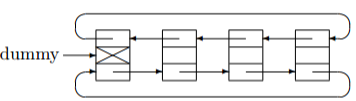# Josephus Problem & Radix Sort Reflection

This post is a reflection on the two problems (MAW 3.13 & 3.10) I have been working on for the past five days.

## Dynamic arrays in C

One of the ways I try out to solve Josephus Problem is to use circular double linked list, which is implemented in ET circularDoubleLinkedListJosephus(ET N, ET M), Inside the function, here is what I try to do initially:

```ET people[N] = {0};
for (i = 0; i < N; i++)
{
people[i] = i + 1;
}
```

I try to make an array of consecutive numbers based upon the input N. However, this way doesn't work in C because compiler has no clue the size of array during compilation phase. This is what people called dynamic array in C. The following two pages offer execellent explanations to dynamic array specific and array in C in general:

Here is what I've done eventually:

```people = calloc(N, sizeof(int));

for ( i = 0; i < N; i++)
{
*(people + i) = i + 1;
}
```

There is one thing to note about calloc. It essentially the same as malloc in terms of allocating a chunk of array. However, unlike malloc, calloc will zero-initlize the chunk, which is quite useful when we work with array of integers. In other words, people = malloc(N*sizeof(int)); is perfectly fine in this case but calloc gives an advantage to have more control on array content, especially useful when we debug.

In MAW, author is kind of in-rush when talks about this section. When comes to implementation, how we deal with header node need to carefully think through. This is what stated in the book:

A popular convention is to have the last cell keep a pointer back to the first. This can be done with or without a header (if the header is present, the last cell points to it) and can also be done with doubly linked lists (the first cell's previous pointer points to the last cell).

Here is my circularly double linked list in picture:In words, our dummy node's Next points to the the first data node and Prev points to the last data node. With this setup, the head of the list can be accessed by dummy.Next and tail by dummy.Prev. In addition, there will never be NULL pointers in the data structure.

## Initialize an array of structs

When implement the radix sort solution, I need to construct an array of buckets, with each bucket is a single linked list with a dummy node. Here is what I do:

```static List
makeEmptyArrayOfNodes(numBuckets)
{
Pos Buckets = malloc(numBuckets * sizeof(struct Node));

int k;
for (k = 0; k < numBuckets; k++)
{
Buckets[k].Next = NULL;
}

return Buckets;
}
```

Here are two points worth noting:

• Pos is struct Node* (can be checked by ptype in GDB) when we allocate an array of struct Node.

• There is difference between -> and .. In K&R Page 131, it says that:

If p is a POINTER to a structure, then p->member-of-structure refers to the particular member.

and . is used to directly access a structure member. In my case, since Buckets[k] with type struct Node, then I need to use .. However, if I want to use ->, I need to use (&Buckets[k])->Next.

## For loop instead of while

I try to experiment different trick when I work on my algo. Here is what I try: use for loop instead of while:

```deleteNode(ET elem, List L)
{
Pos dummyL = L->Next;
Pos dummyPrev = L;

for(; dummyL != NULL; dummyPrev = dummyL, dummyL = dummyL->Next)
{
if (dummyL->Element == elem)
{
Pos tmp = dummyL;
dummyPrev->Next = dummyL->Next;
free(tmp);
return;
}
}
}
```

## Use system implementation if find, otherwise use my own version

I'm trying to use fls inside int cyclicShiftJosephus(int N, int M), which return the last (most significant) bit set in value and return the index of that bit. However, not all system has fls shipped by default. So, I implement my own version. But, I would prefer the program to use system version if it can find one. Otherwise, use mine.

One solution is to use #ifndef with the structure looks like

```#ifndef fls
int fls(int mask) { ... }
#endi
```

Another solution is to use weak symbol. However, this solution may not be portable. Then, it looks something like this

```int  __attribute__((weak)) fls(int mask){ .. }
```

If system fls is defined as strong, my fls implementation will be overridden.